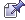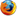###Author Topic: Calculator N+ (Open source) - Math Solver for Android does complex functions.  (Read 444 times)

0 Members and 1 Guest are viewing this topic.

#### Software Santa

•• Posts: 4615
• OS:
•Mac OS X 10.9
• Browser:
•Firefox 60.0##### Calculator N+ (Open source) - Math Solver for Android does complex functions.
« on: January 27, 2019, 05:04:57 PM »
Calculator N+ (Open source) - Math Solver for Android does complex functions. Ad Free. Permissions Free.

Quote
The Calculator provides the following features:

ALGEBRA
1. Computer science
2. Solving the equation:
3. Solve system equations
4. Graphing
5. Cartesian geometry sand(Viet Nam Education)
6. Unit Conversions
8. Simplify expressions
9. Polynomial factorization.
10. Binomial expansion Newton
11. Matrix: evaluate matrix step by step

ANALYTICS
1. Derivative
2. Antiderivative
3. Definite integrals
4. Find the limit of the sequence, function

TRIGONOMETRIC
1, Trigonometric expand: sin(2x) -> 2sin(x)cos(x)
2. Trigonometric reduce: 2sin(x)cos(x) -> sin(2x)
3. Trigonometric to exponent: sinh(x) -> (e^x-e^(-x))/2

DETERMINATION OF STATISTICS
1. Combination
2. Permutation

SOME OTHER FEATURES
1. Prime factors
2. Modulo
3. Catalan number
4. Fibonacci number

The Calculator does not show the steps to solve the equations, system of equations, derivative... but only for the final result.
Enter sin(30°) to calculate sine Degree mode

The computer can work in two modes:
- Decimal mode: 0.12312312323
- Mode fractions: for results with arbitrary precision, for example 9^99999

Any suggestions on the application please send mail: Tranleduy1233(at)gmail.com.

See in github https://github.com/tranleduy2000/ncalc

Software Santa first Opened on January 1st, 2007
Software Santa's Speedy Site is Proudly Hosted by A2 Hosting.
The best present of all: will be The Second Coming
of Christ on Christmas Day of 2020.

Welcome Visitor:

 @ MEMBER OF PROJECT HONEY POTSpam Harvester Protection Networkprovided by Unspam

Software Santa Welcome Page